## In a glance

This module calculates the costs of heat supply in buildings via decentral heat supply technologies. Inputs to the module are investment costs, O&M costs, energy prices, the hourly load profile of heat demand as well as depreciation time and interest rate. Furthermore, the composition of buildings and renovation states of the buildings in the analysed area are required. The outputs are heat supply costs of various decentral heat supply technologies for the defined buildings and price assumptions as well as reference decentral supply costs for the analysed area and related CO2 emissions.

To Top

## Introduction

This calculation module calculates the levelized cost of heat (LCOH) for various decentral heat supply technologies for supplying heat to a user-defined building.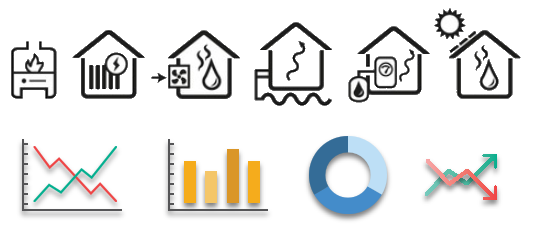The technologies are for example as follows:

• Heat pump air-to-air, air-to-water and Brine-to-water,
• Electric heater,
• Bio-oil boiler,
• Oil boiler,
• Biomass automatic and manual,
• Wood-stove,
• Natural gas,
• Solar thermal

To Top

## Inputs and outputs:

### Inputs

#### Default input data

Part of the input data comes from the Hotmaps default data sets. These include:

• NUTS Raster
• Building stock (NUTS0 Level): useful energy demand by building class and construction period
• Financial Data - Heating Systems (NUTS0 Level)
• Financial Data - Energy Carrier Prices (NUTS0 Level)

To Top

#### Manual input data

The following input data should be entered manually after the selection of an area:

• Building age,
• Interest rate,
• Gross floor area,
• Building Type,
• Year (for finance data),
• Savings in space heating,
• Emission factors per energy carrier.

IMPORTANT NOTE In Hotmaps biomass emissions are not assumed zero because Hotmaps is a tool aimed at supporting the ongoing energy transition. Considering biomass as a carbon-neutral source of energy implies that burning biomass reduces the current total emissions compared to burning fossil fuels, which is not true. The storage of carbon dioxide in trees happens over decades, while its release in the atmosphere takes place all at once. Therefore, it can be concluded that the net carbon balance of biomass is zero in the long run, but not in the short run and climate change mitigation measures need to bring results in reducing carbon emissions in the short run.

To Top

### Outputs:

#### Indicators:

Indicators are composed of a summary of input parameters and calculated values:

• Corresponding NUTS Code: NUTS3 Code of the selected region (when using hectare level or LAU level),
• entered savings in space heating,
• entered gross floor area,
• entered year,
• entered interest rate,
• entered building age,
• entered building type,
• annual useful energy demand of the building,
• Sector,
• Used Building type for each the financial data.

To Top

#### Graphs:

• LCOH
• OPEX
• CAPEX
• Energy Costs
• CO2 Emissions
• Thermal Efficiency
• Final Energy Demand
• Total Costs
• Annuity factor

To Top

## Methodology:

Based on the selected region the corresponding NUTS0 and NUTS2 Regions are determined.

Then, for the selected building type and building age and NUTS0 region the specific useful energy demand is selected and the annual heat demand calculated by multiplying it by the gross floor area.Based on the annual heat demand of the building and the entered savings in space heating the heat load is calculated with the use the heat load profiles.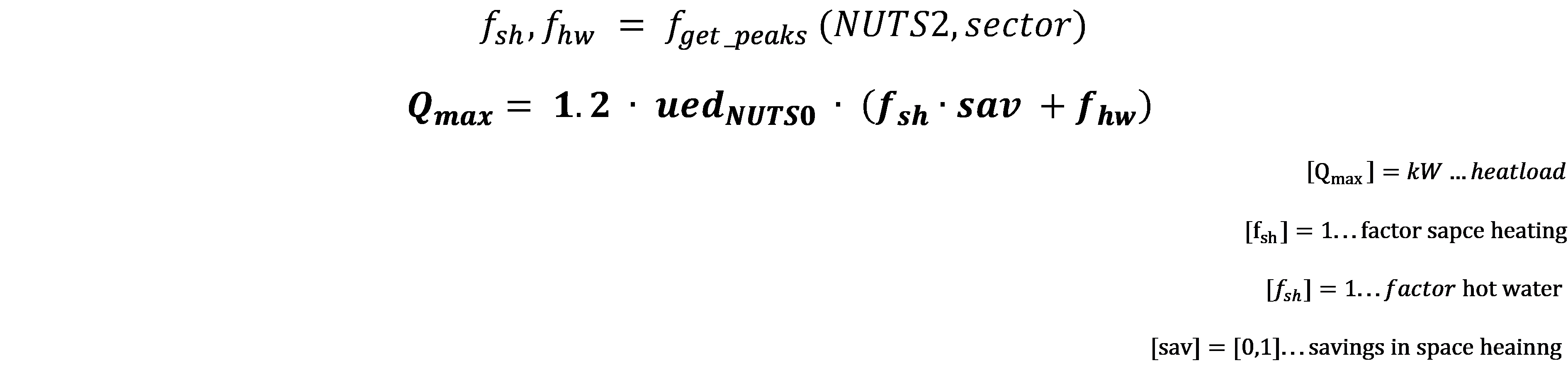With the heat load, the annual heat demand and the selected year the investment costs and operational costs and fuel costs for each heating technology are determined.

The specific investment and operational costs are assumed to have an exponential behavior:Based on the country, year and building type of the entered inputs the factors for each technology are figured out: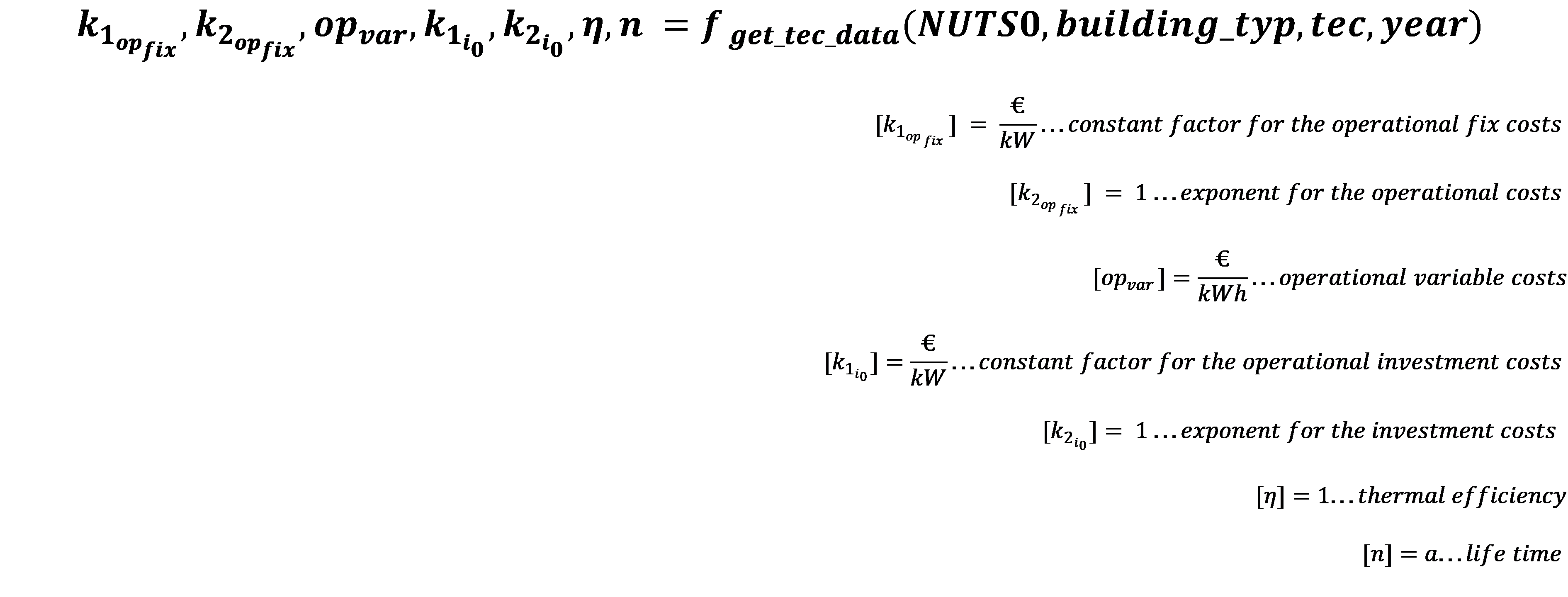With the factors determined, the absolute costs can be calculated as follows:For the fuel costs, the price data of the energy carriers are needed.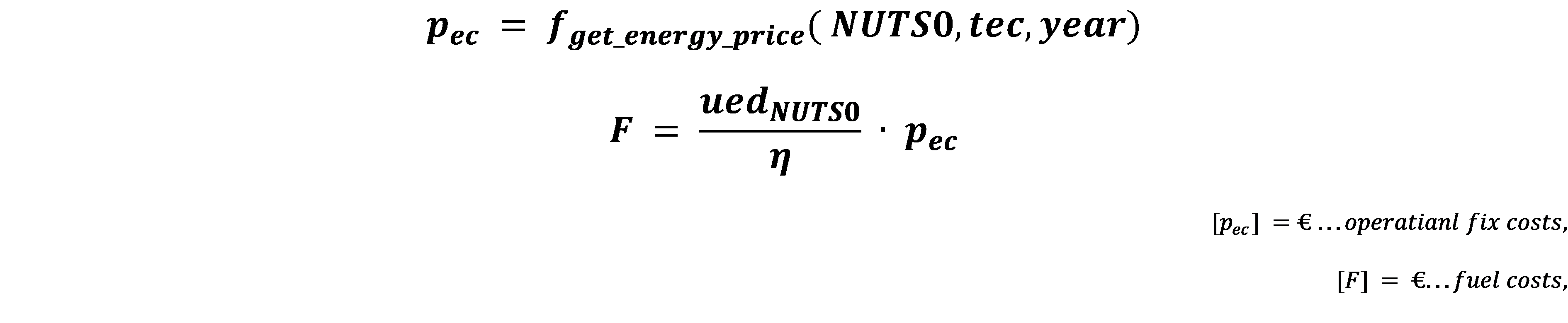Now we have all the needed data and can calculate the levelized cost of heat.

The levelized cost of heat for each heating system is calculated as the net present value of the cash flow and the heat generation over the lifetime.

The levelized cost of heat is given by: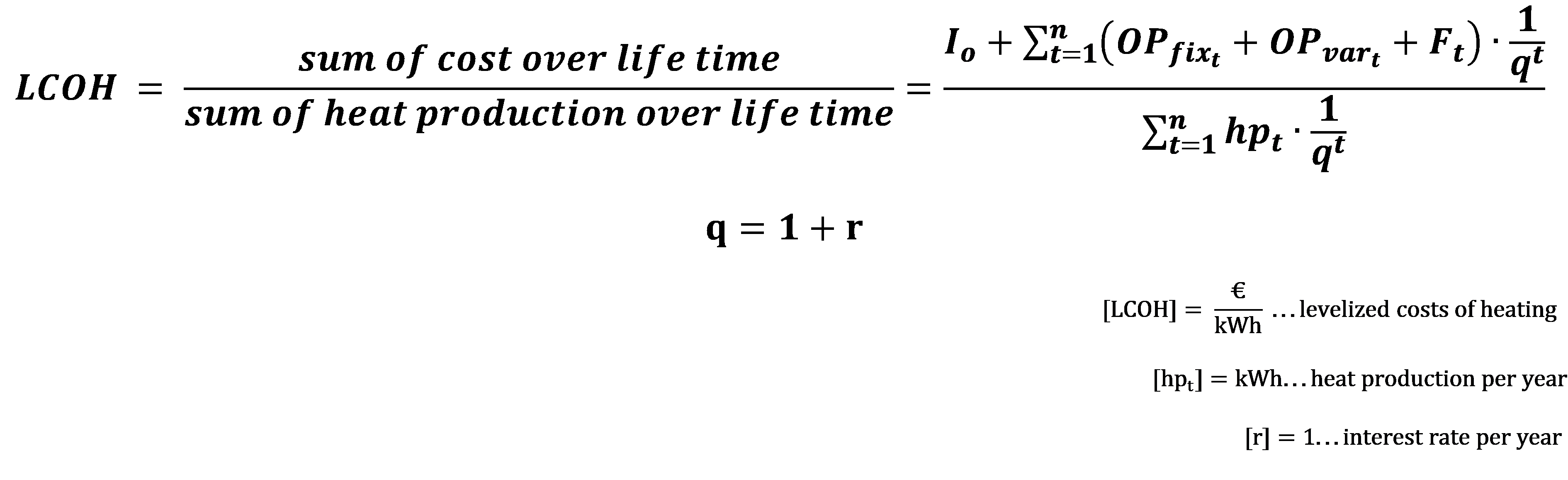In this calculation the yearly costs and the yearly heat production (useful energy demand) is assumed to be constant over the lifetime and thus the summations transform into a geometric series and the transforming factor is called the annuity factor α. A graphical representation of this transforming process is shown in the figures below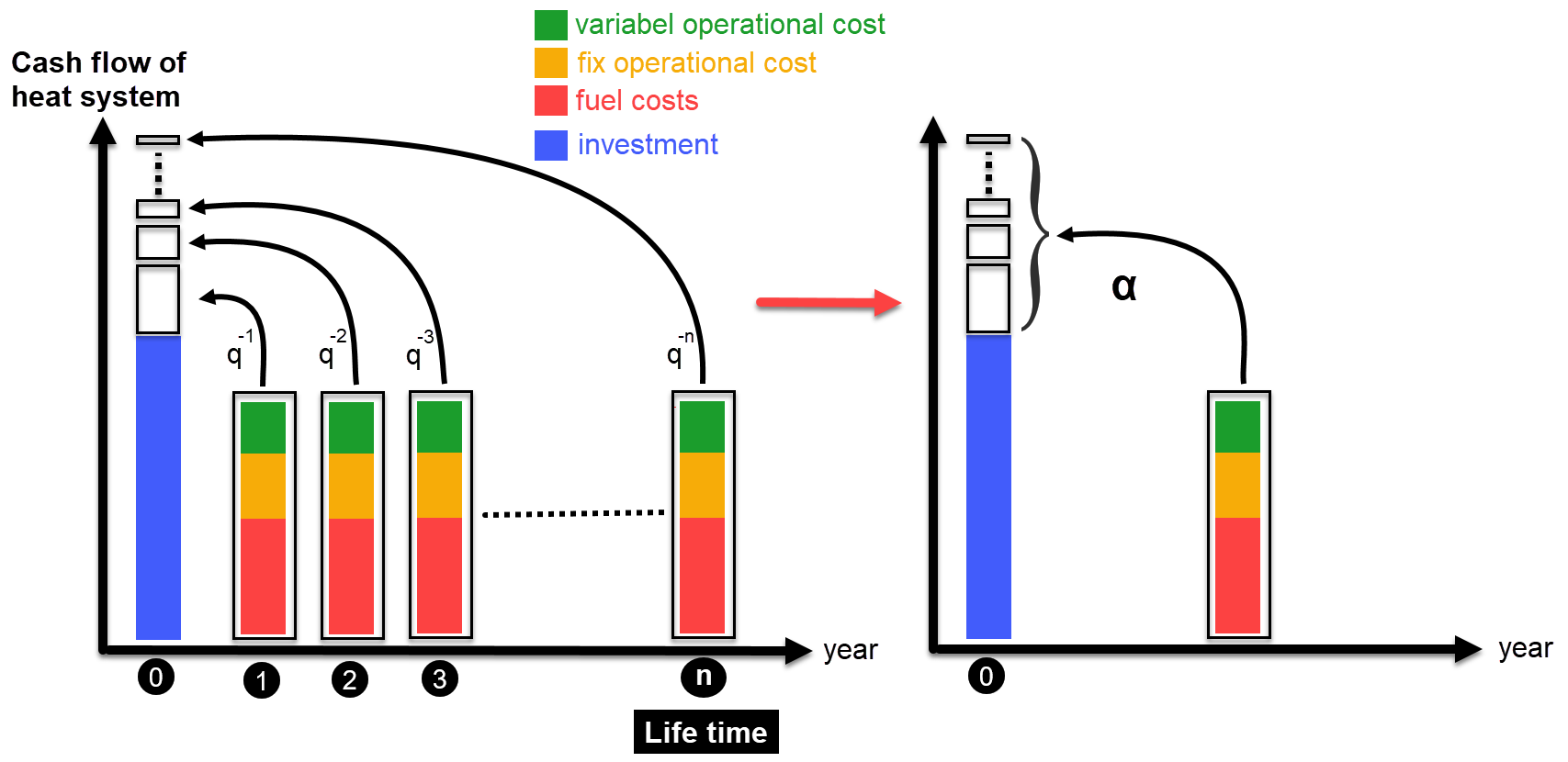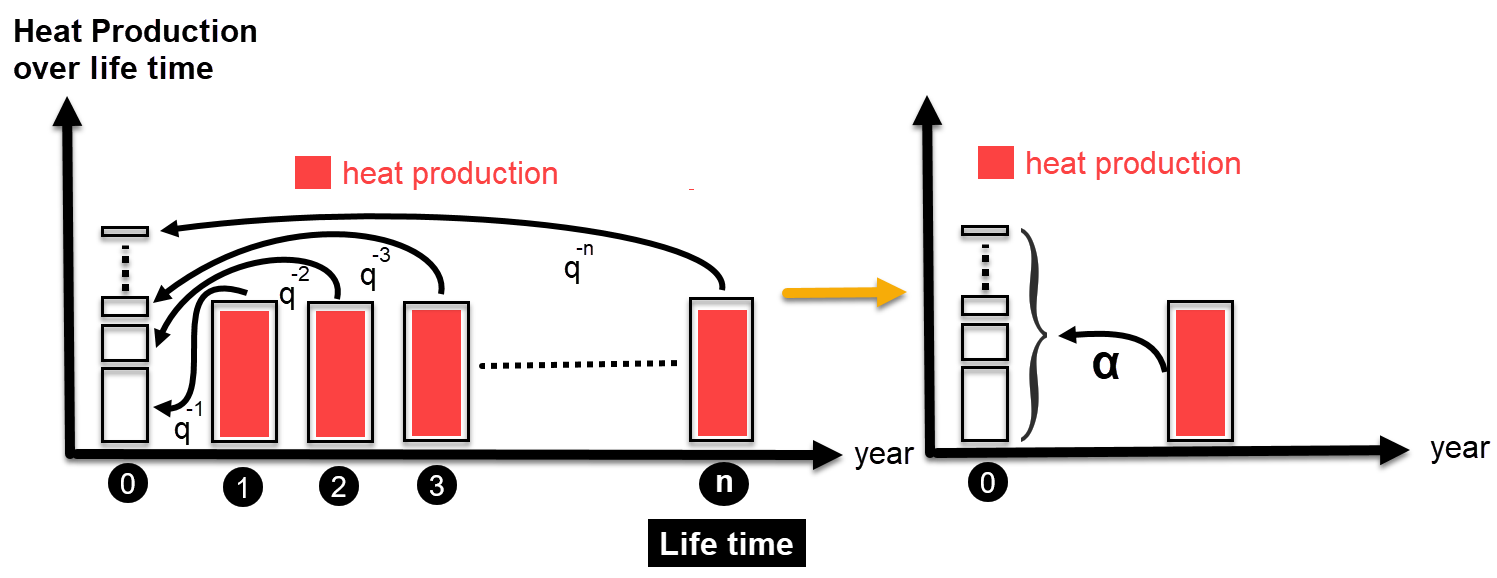This assumption simplifies the calculation and the LCOH is given by the following formula:To Top

## GitHub repository of this calculation module

Here you get the bleeding-edge development for this calculation module.

To Top

## Sample run

### Test Run

#### Default Input Parameters#### Output Indicators With Default Input Parameters#### Output Charts With Default Input ParametersTo Top

## How to cite

Jeton Hasani in Hotmaps-Wiki, CM Decentral heating supply (November 2019)

To Top

## Authors and reviewers

To Top

To Top
To Top